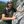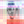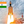0%
0%

# Arduino Wattmeter - Voltage and Power Consumption

A device can be utilized to measure the power consumed. This circuit can also act as a Voltmeter and Ammeter to measure voltage and current.

Similar projects worth following
932 views
We design a circuit using Arduino which will act as Voltmeter as well as Ammeter to measure voltage and current.

Building your own meters not only carries down the cost of testing but also provides us room to facilitate the process of testing.

Working of the Project

From the sensor part, there are two sections that are reliable for measuring voltage and current. For measuring the voltage, a voltage divider circuit is executed using a 10KΩ and a 2.2KΩ Resistor.

With the help of these resistors, you can easily measure voltages up to 24V. These resistors also support us in taking the voltage range to 0V – 5V, which is the normal range on which Arduino works.

In order to measure the current, we have to change the current values to conventional voltage values. As per Ohm’s Law, the voltage drop across a load is proportional to the current. Hence, a small shunt resistor is arranged with respect to the load. By estimating the voltage across this resistor, we can calculate the current.

We have used LM358 Op-Amp in Non-Inverting Amplifier Mode to magnify the values provided to Arduino. The voltage divider network for the feedback control includes a20KΩ Resistor and 1KΩ Resistor. These resistors offer a gain of approximately 21.

After Implementation Arduino Wattmeter

• 1 × Arduino Uno
• 1 × LCD 16X2
• 1 × Linear Regulator (7805)
• 1 × Single Turn Potentiometer- 10k ohms
• 1 × Ceramic Disc Capacitor, 0.1 µF
• 1
Run a Program

#include

const int rs = 2, en = 4, d4 = 9, d5 = 10, d6 = 11, d7 = 12;
LiquidCrystal lcd(rs, en, d4, d5, d6, d7);
float Voltage = 0.0;
float Current = 0.0;
float Power = 0.0;
void setup()
{
lcd.begin(16, 2);
Serial.begin(9600);

lcd.print(" Arduino ");
lcd.setCursor(0, 1);
lcd.print(" Wattmeter ");

delay(2000);
lcd.clear();

}

void loop()
{

Voltage = Voltage * (5.0/1023.0) * 6.46;
Current = Current * (5.0/1023.0) * 0.239;

Serial.println(Voltage);
Serial.println(Current);

Power = Voltage * Current;

Serial.println(Power);

lcd.setCursor(0, 0);
lcd.print("V="); lcd.print(Voltage);
lcd.print(" ");
lcd.print("I=");lcd.print(Current);
lcd.setCursor(0, 1);
lcd.print("P="); lcd.print(Power);
delay(1000);
}

Share

## Similar Projects

Project Owner Contributor

### Capacitive discharge spot welderkevarek

Project Owner Contributor

### Spin/Drive: Automatic potentiometer tracerBen Holmes

Project Owner Contributor

### Arduino based simple Watt-meter shieldSagar 001

# Does this project spark your interest?

Become a member to follow this project and never miss any updates